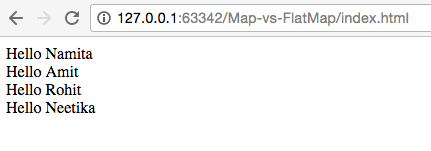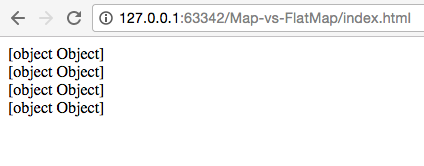# Map vs FlatMap

Anyone who has worked upon/read about RXJS must be aware about various operators that this library includes, some of them are:

1. 0f - It simply converts a list of arguments into an Observable sequence.
2. from - Creates an Observable sequence from an array or an object that can be iterated.
3. map - Transforms each element of the Observable sequence. Can be considered similar to map function of Array.
4. subscribe - This operator is basically the connecting point between an Observer and Observable. An Observer receives item/error/completion notification from Observable using the subscribe operator. A cold observable would start emitting value only when an observer subscribes to it.

The above ones are like most commonly used and you would get to know many new ones.

Well, I encountered a situation where I had Observable of Observables and I wanted a single stream out of them and to solve this I got introduced to another interesting operator:

flatMap - It basically merges an observable sequence of observable sequences into a single observable sequence.

So, let’s take a sample snippet to see how the it works. We have an array of visitors as given below:

``````let visitors = [
"Namita",
"Amit",
"Rohit",
"Neetika"
];
``````

Now, we want this array to be converted into an Observable sequence, so it can be done something like:

``````let source = Rx.Observable.from(visitors)
.map(x => 'Hello ' + x);
``````

We will now have to subscribe to this sequence:

``````source.subscribe(x => document.getElementById('flatMap').innerText += x + "\n");
``````

And view would look like this:But what we wanted to see was how to work with observable of observable sequence, so for that let’s make some changes as given below:

``````let source = Rx.Observable.from(visitors)
.map(x => Rx.Observable.of('Hello ' + x));
``````

… and our view would look something like this:So how to fix this up? Well, now we’ll have to use our flatMap operator as given below:

``````let source = Rx.Observable.from(visitors)
.flatMap(x => Rx.Observable.of('Hello ' + x));
``````

and now one can simply subscribe to it as we were doing earlier and our view as per our expectations:So what’s the exact difference between map and flatMap:

map transforms items emitted by an Observable by applying a function to each item whereas flatmap:

1. Applies a specified function to each emitted item and this function in turn returns an Observable for each item.
2. flatMap then merges all these sequences to make a new sequence.

So let’s make a small ASCII marbel to make our understanding more clear:

``````----Namita---Amit---Rohit---Neetika----- //Input Stream
.map(x => 'Hello ' + x);
---Hello Namita---Hello Amit---Hello Rohit---Hello Neetika--- //Map's function result
``````
``````----Namita---Amit---Rohit---Neetika----- //Input Stream
.flatMap(x => Rx.Observable.of('Hello ' + x))
--Hello Namita--     //transforming each input element into an Observable
--Hello Amit--
--Hello Rohit--
--Hello Neetika--
---Hello Namita---Hello Amit---Hello Rohit---Hello Neetika--- // Flatmap's final result
``````

There is also another operator named as .mergeAll which we can use with map when we are in observable of observables situation instead of directly using flatMap. RxJS has numerous operators and hopefully this learning voyage will take us to each one of them.. till then happy learning!